Next: Magnetostatic Fields Up: Electrostatics in Dielectric Media Previous: Dielectric Liquids in Electrostatic

# Exercises

1. Starting from Equation (201), derive the result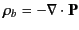.

2. Consider an electron of charge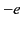moving in a circular orbit of radius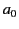about a chargein a field directed at right angles to the plane of the orbit. Show that the polarizability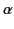is approximately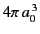.

3. A point chargeis located in free space a distancefrom the center of a dielectric sphere of radius(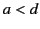) and dielectric constant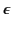. Find the potential at all points in space as an expansion in spherical harmonics. Calculate the rectangular components of the electric field in the vicinity of the center of the sphere.

4. A dielectric sphere of radiusand dielectric constant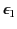is imbedded in an infinite dielectric block of dielectric constant. The block is placed in a uniform electric field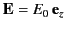. In other words, if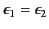then the electric field would be. Find the potential both inside and outside the sphere (assuming that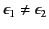), and the distribution of bound charges on the surface of the sphere.

5. An electric dipole of moment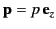lies at the center of a spherical cavity of radiusin a uniform dielectric material of relative dielectric constant. Find the electrostatic potential throughout space. Find the bound charge sheet density on the surface of the cavity.

6. A cylindrical coaxial cable consists of a thin inner conductor of radius, surrounded by a dielectric sheath of dielectric constantand outer radius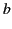, surrounded by a second dielectric sheath of dielectric constantand outer radius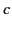, surrounded by a thin outer conductor. All components of the cable are touching. What is the capacitance per unit length of the cable?

7. A very long, right circular, cylindrical shell of dielectric constantand inner and outer radiiand, respectively, is placed in a previously uniform electric field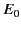with its axis perpendicular to the field. The medium inside and outside the cylinder has a dielectric constant of unity. Determine the potential in the three regions, neglecting end effects. Discuss the limiting forms of your solutions for a solid dielectric cylinder in a uniform field, and a cylindrical cavity in a uniform dielectric.

8. Suppose that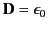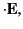where the dielectric tensor,, is constant (i.e., it is indepedent of). Demonstrate that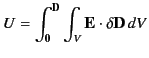can only be integrated to give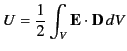ifis symmetric. (Incidentally, because we generally expect a dielectric system to be conservative, this proves thatmust be a symmetric tensor, otherwise the final energy of a dielectric system would not be independent of its past history.)

9. Show that for an electret (i.e., a material of fixed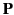) the integral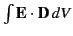over all space vanishes.

10. Two long, coaxial, cylindrical conducting surfaces of radiiand(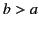) are lowered vertically into a liquid dielectric. If the liquid rises a mean height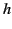between the electrodes when a potential difference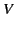is established between them, show that the susceptibility of the liquid iswhere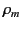is the mass density of the liquid,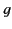the acceleration due to gravity, and the susceptibility of air is neglected.Next: Magnetostatic Fields Up: Electrostatics in Dielectric Media Previous: Dielectric Liquids in Electrostatic
Richard Fitzpatrick 2014-06-27